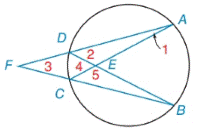Chapter 6.2, Problem 2E### Elementary Geometry for College St...

6th Edition
Daniel C. Alexander + 1 other
ISBN: 9781285195698

#### Solutions

Chapter
Section### Elementary Geometry for College St...

6th Edition
Daniel C. Alexander + 1 other
ISBN: 9781285195698
Textbook Problem
150 views

# Given: m D C ⌢ = 30 ° and m D A B C ⌢ is trisected at points A and B Find: a) m ∠ 1             d) m ∠ 4 b) m ∠ 2           e) m ∠ 5 c) m ∠ 3To determine

(a)

To find:

m when mDC=30° and mDABC is trisected at points A and B.

Explanation

Corollary:

The measure of an angle formed by a tangent and a chord drawn to the point of tangency is one-half the measure of the intercepted arc.

Calculation:

m1 (DAC)

mDABC is trisected at points A and B.

DA=AB=BC

mAB+mBC+mCD+mDA=360°mAB+mAB+30°+mAB=360°30°+3m<

To determine

(b)

To find:

m2 (ADB) when mDC=30° and mDA=mAB=mBC=110°.

To determine

(c)

To find:

m3 (AFB) when mDC=30° and mDA=mAB=mBC=110°.

To determine

(d)

To find:

m4 (DEC).

To determine

(e)

To find:

m5 (CEB).

### Still sussing out bartleby?

Check out a sample textbook solution.

See a sample solution

#### The Solution to Your Study Problems

Bartleby provides explanations to thousands of textbook problems written by our experts, many with advanced degrees!

Get Started

#### Find more solutions based on key concepts